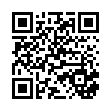# Bira vK .pdf

### File information

Title: Diapositive 1
Author: rowley

This PDF 1.5 document has been generated by Acrobat PDFMaker 10.1 for PowerPoint / Adobe PDF Library 10.0, and has been sent on pdf-archive.com on 11/10/2017 at 16:47, from IP address 134.158.x.x. The current document download page has been viewed 510 times.
File size: 1.14 MB (29 pages).
Privacy: public file

### Document preview

UNITARITY
AND
DISCRETE SCALE INVARIANCE

U. van Kolck
Institut de Physique Nucléaire d’Orsay
and

University of Arizona
Supported by CNRS and US DOE
1

Outline
 What is essential?
 Pionless EFT

with
S. König,
H.W. Grießhammer
&amp; H.-W. Hammer

 Unitarity: light nuclei
 Unitarity: bosonic clusters
 Unitarity: matter
 Conclusion

plus
B. Bazak
&amp; M. Eliyahu

S. Gandolfi,
J. Carlson,
&amp; S.A. Vitiello

L. Contessi,
A. Lovato,
F. Pederiva,
A. Roggero
&amp; J. Kirscher

What is essential in nuclear physics?
approach

1) Describe NN precisely to some high energy
2) Append 3N forces as needed
Lost in details:
e.g., NLO, N2LO, N3LO, N4LO, …

Here

1) Describe NN and 3N approximately
2) Treat everything else in perturbation theory
cf. atomic systems in QED

“simplicity emerging
from complexity”

Expansion
around unitarity

König, Grießhammer,
Hammer + v.K. ’15 ’16
König ’16
vK ‘17

A=2

T2 (k  =
R −1 )

−1

m

r2 2 P2 4
 −1

+

+
+

a
i
k
k
k
 2
 + (l &gt; 0)
2
4

scattering
length

unitarity
limit

(

a2−1 ≈ mB2 → 0

−1
2

T2 a

−1

R  mπ

a2,=I
1

S0

shape
parameter

r2
P
 R, 2  R 3 ,  typically
2
4

 1


−1 
=
kR
( ik ) 1 +   , kR  
m
 ka2


−1

)

unitarity window

nucleons

effective
range

a2,=I

a2,=I

1,=
I3

0 mπ

1,=
I3

+1mπ

1,=
I3

−1mπ

no parameter!

−1

“universality”

 0.06

−1

−1

−1

− a2, I =
 0.12
1,=
I 3 0 mπ
−1

− a2, I =
 0.02
1,=
I 3 0 mπ

3

S1

a2, I =0 mπ

−1

 0.26

unitarity limit

unitarity
limit

mπ∗ ( M QCD )

Feshbach resonance

mπ  140 MeV

MIT webpage

Chiral EFT,
(incomplete) NLO

Beane, Bedaque,
Savage + v.K. ’02
Beane + Savage ’03’04
Epelbaum, Glöckle
+ Meißner ‘03

or “accidentally”, e.g.

a2  mπ−1  r2

4He

a2  lvdW  r2

atoms

ground
states

Q A  2 mN B A A

A

QA mπ

2
3
4
5
6

0.3
0.5
0.8
0.7

56

Q2  a2,−1I =0 ≡ ℵ1  M lo

3

S1 unitarity

König, Grießhammer,
Hammer + v.K. ’16
v.K. ‘17

QA≥3  M lo

0.7

0.9

König, Grießhammer,
Hammer + v.K. ’15
König ’16

1

S0 unitarity

a2,=I

−1
1,=
I3 0

≡ ℵ0  M lo

−1

−1

−1

−1

a2, I =
− a2, I =
 α mN  ℵ0  M lo
1, I 3 =
+1
1, I 3 =
0
a2, I =
− a2, I =
 md − mu  ℵ0
1, I 3 =
1, I 3 =
0
−1

Effective Field Theory

momentum
scales

M hi

Λ

UV regulator

T

M lo

non-analytic functions,
from solution of dynamical eq.
(e.g. Lippmann-Schwinger)

arbitrary

Q

(ν )

ν

 Q Q (ν )  Λ M lo
 Q 
(ν )
(Q  M lo  M hi ) ∝ ∑ 
, ; γi 
,
 F 
ν = 0  M hi 
 M lo M hi
 M lo Λ
ν

ν

N LO
(unfortunately not the usage
by potential modelers)

RG invariance
(absent in “chiral potentials”)

Q 
Λ ∂T
= ν 
(ν )
T
∂Λ
 M hi Λ 
(ν )

c

ν +1

model independent

 Qν +1 Qν +1 
+   ν +1 , ν 
 M hi M hi Λ 


 


“low-energy
constants”

controlled
(OTHERWISE, NOT ERROR ESTIMATE)
to minimize cutoff errors, Λ &gt; M hi

for realistic error estimate, Λ ∈ [ M hi , ∞ )
(OTHERWISE, SENSITIVE TO HIGH-MOM DETAILS)

Pionless EFT
Q  M lo  M hi  mπ

• d.o.f.: nucleons
• symmetries: Lorentz, P, T, B, SU(3)c, U(1)em
(trivial)

dt
3
d
∫ 2m ∫ r

S EFT
most general
action

 +
∂ 2
ψ  2im + ∇ ψ
∂t

 
projector on isospin I
+ +
− 4π ∑ C0 I ψ ψ PI ψψ
I = 0,1

(4π ) 2
D0 ψ +ψ +ψ + ψψψ

3
more derivatives,
+ } more bodies,
isospin violation

Universality:
first orders
apply also to
neutral atoms

mπ → 1 lvdW

4
vdW

l
where V ( r ) =

+
6
2mr

Bedaque, Hammer
+ v.K. ’99’00
Bedaque, Braaten
+ Hammer ’01

Now,
two expansions:
ℵ1 M lo ≡ Q2 Q3  0.4
M lo M hi  Q3 mπ  0.5

around two-body unitarity
standard Pionless EFT

similar:

ℵ1  M lo2 M hi

For simplicity, also:

starts at NLO

α mN  ℵ0  M l2o M hi
md − mu  M lo3 M h2i

starts at NLO
starts at N2LO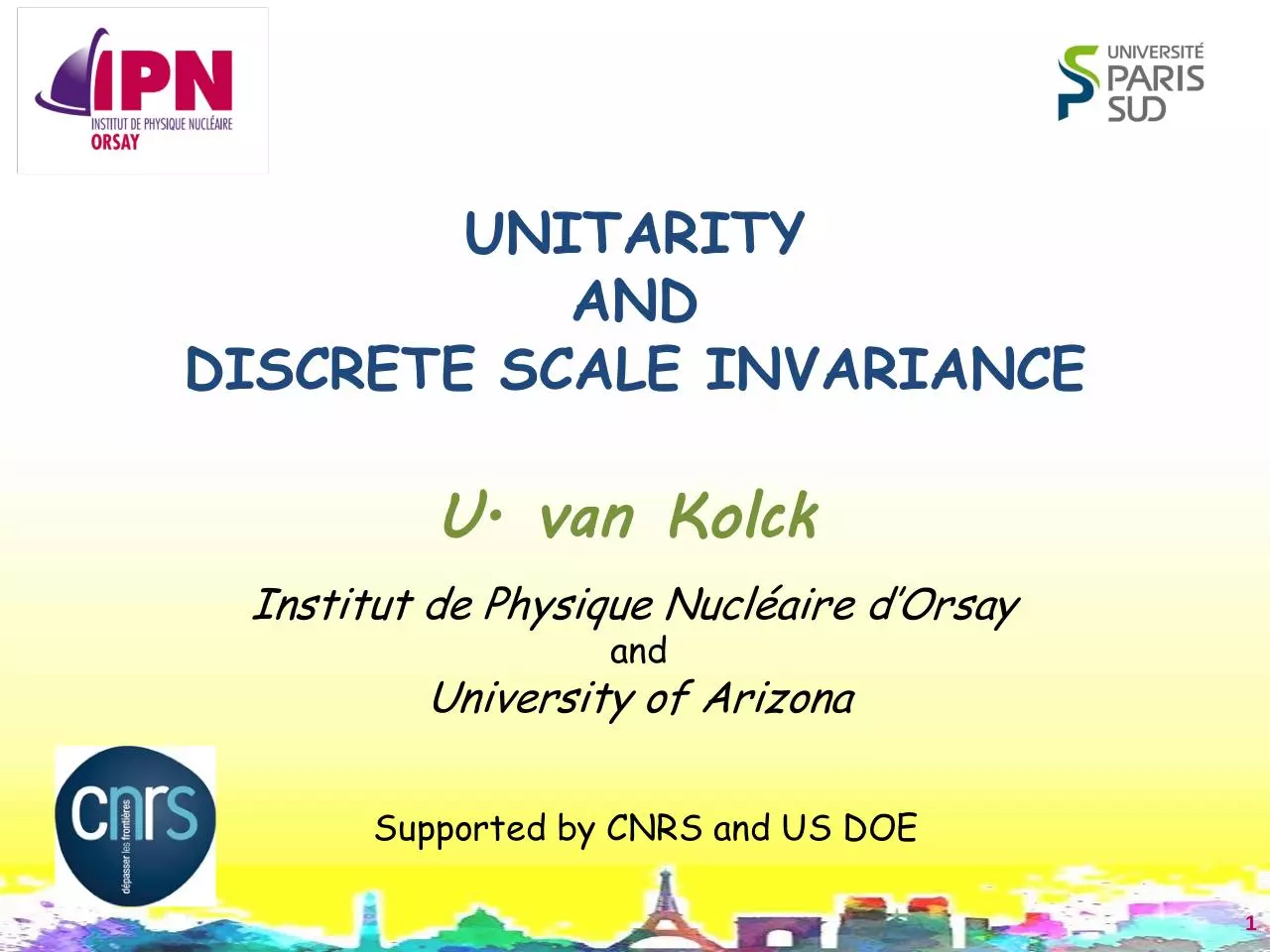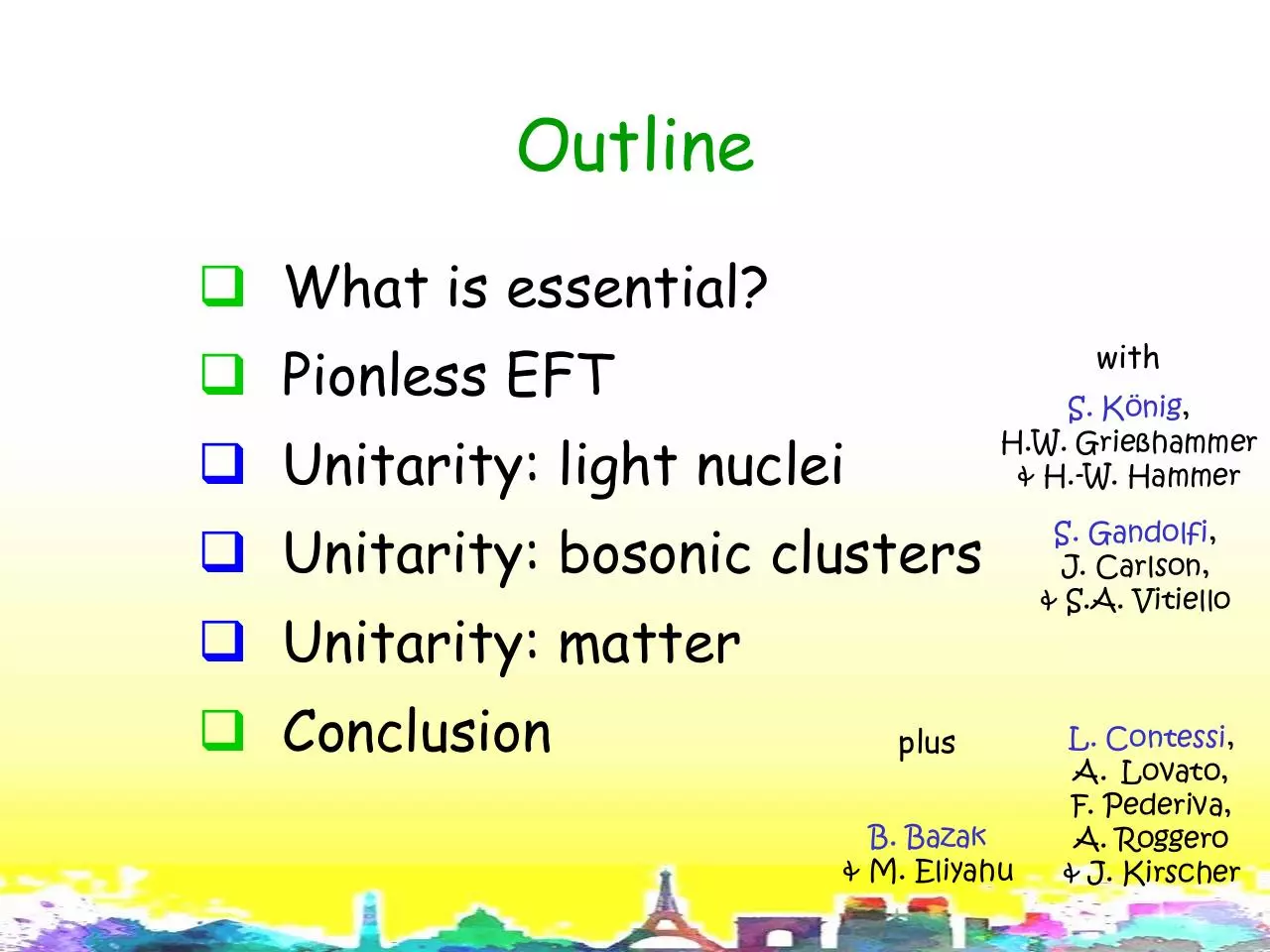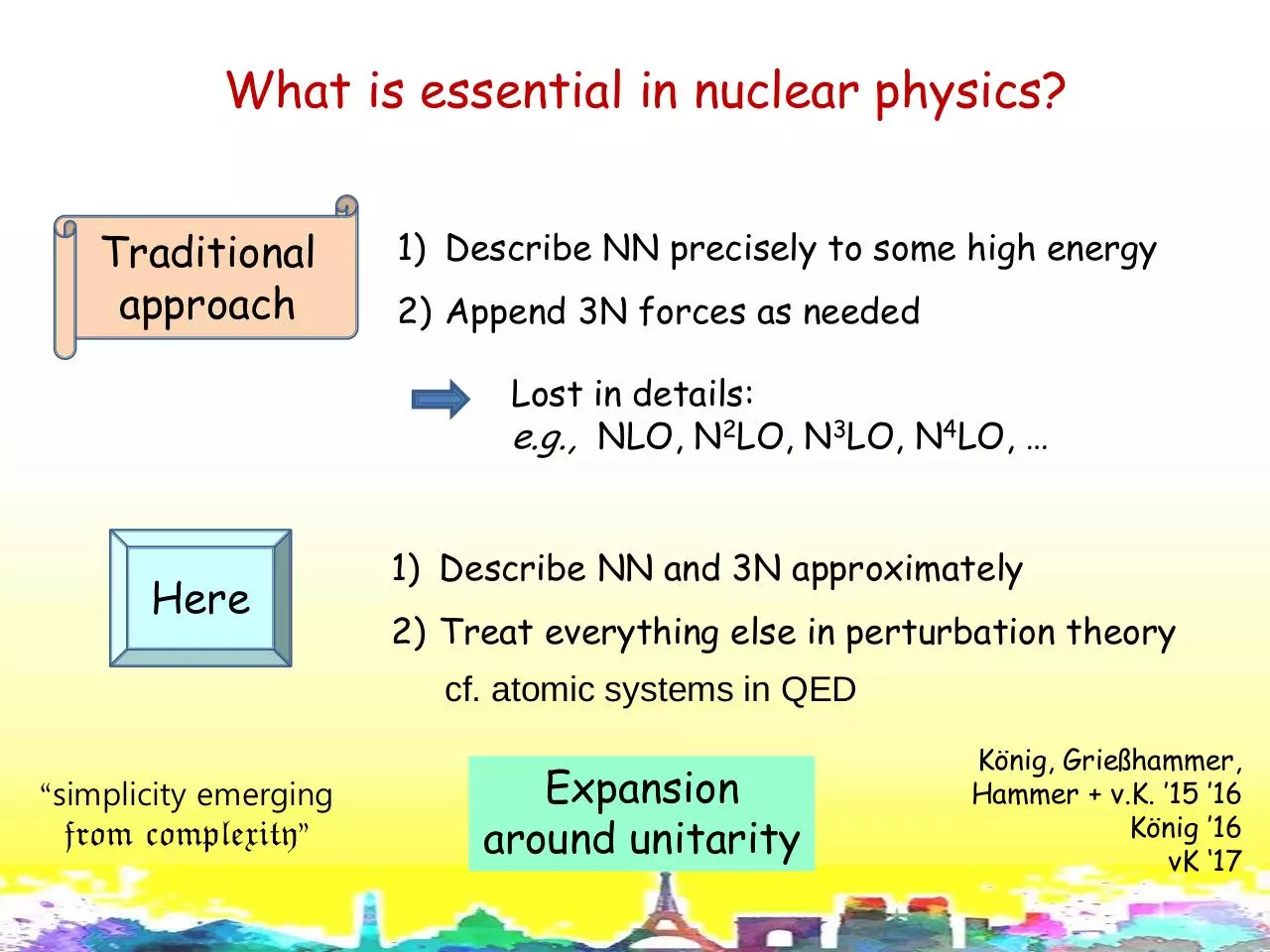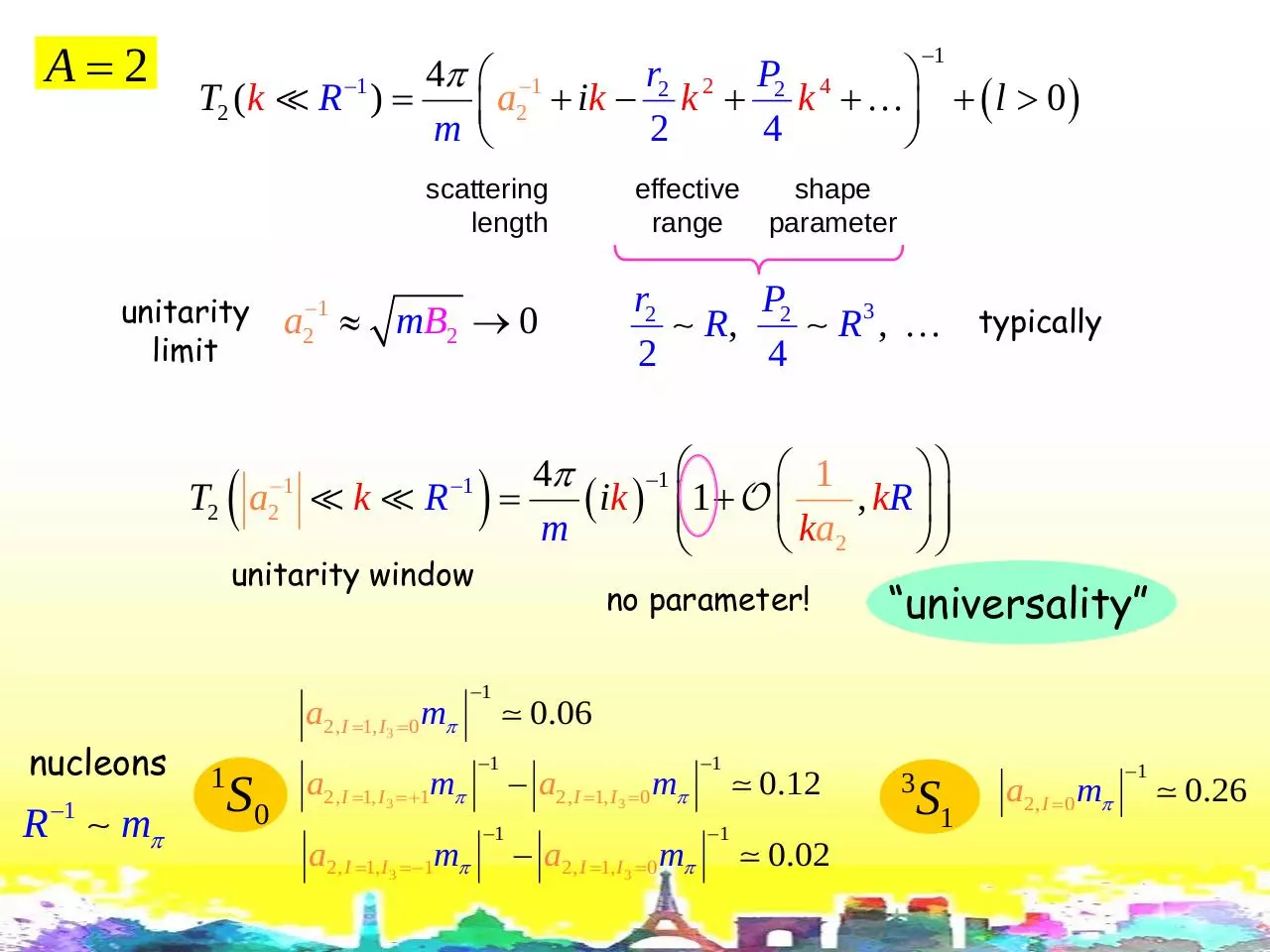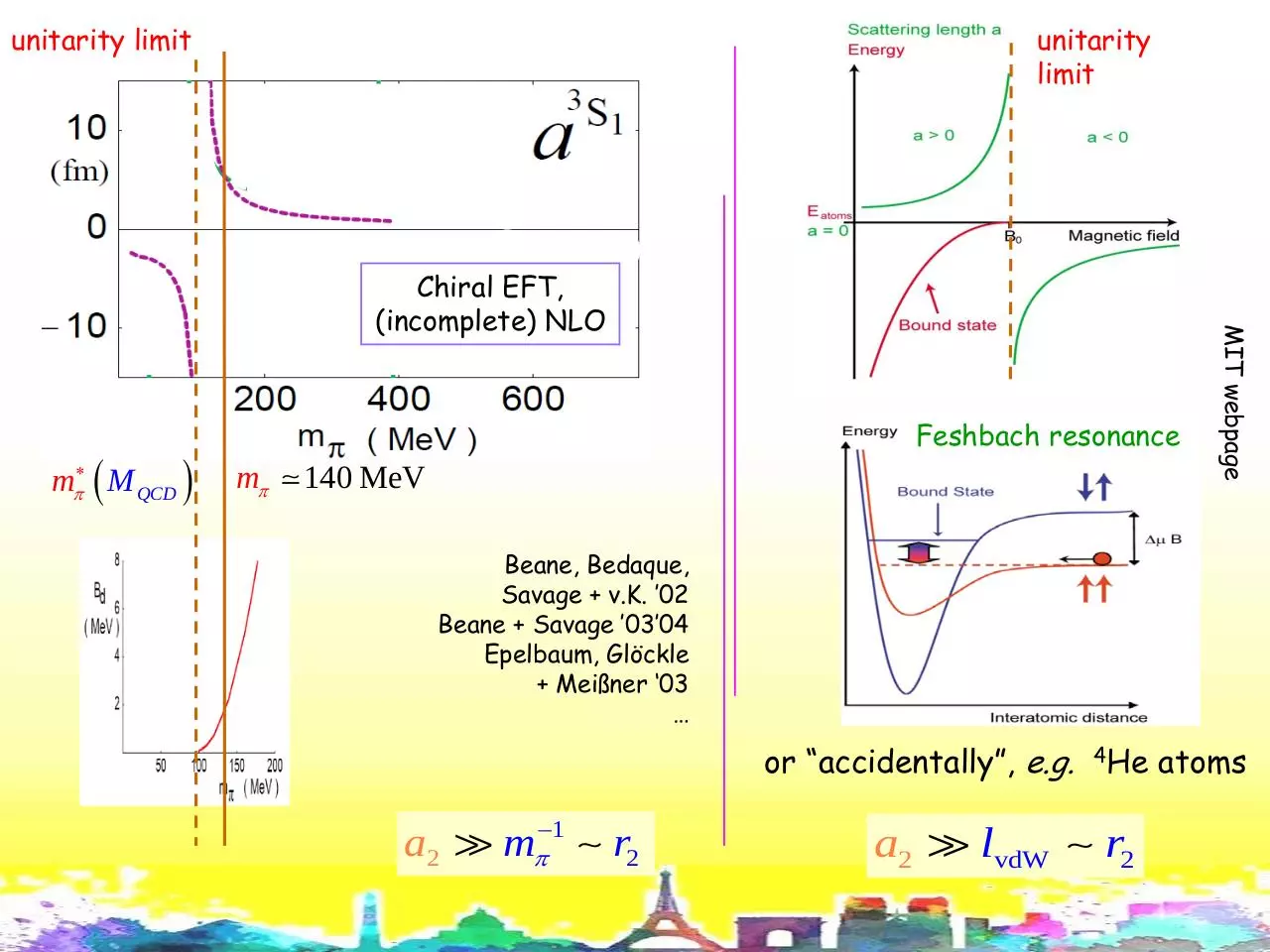Bira vK.pdf (PDF, 1.14 MB)

### Share on social networks

#### HTML Code

Copy the following HTML code to share your document on a Website or Blog